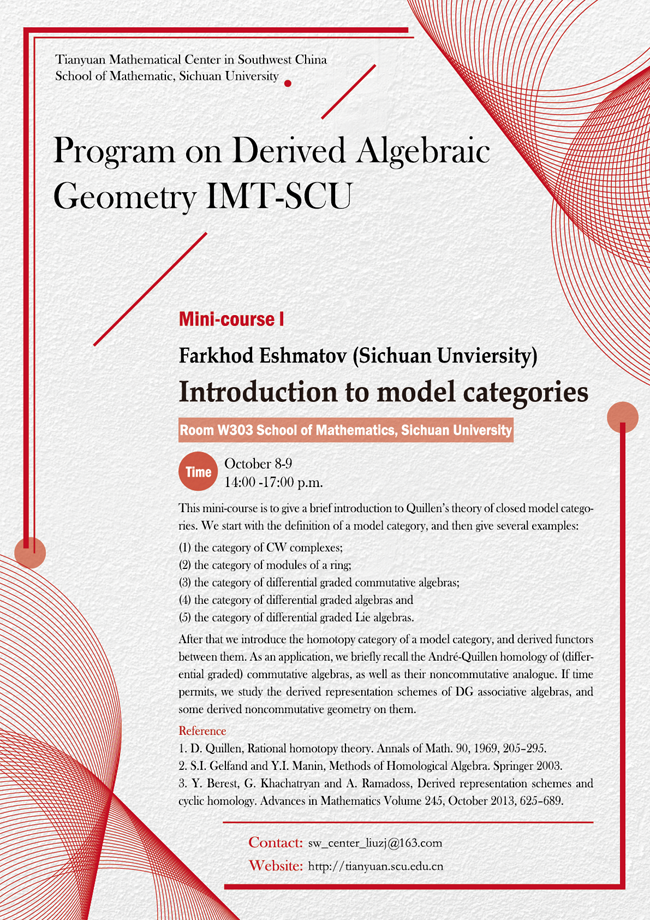2019 Thematic Program (IV)

### Introduction to model categories

#### Venue: W303  School of Mathematics, Sichuan University#### SPEAKER

Farkhod Eshmatov (Sichuan University)

#### ABSTRACT

This mini-course is to give a brief introduction to Quillen’s theory of closed model categories. We start with the definition of a model category, and then give several examples:

(1) the category of CW complexes;

(2) the category of modules of a ring;

(3) the category of differential graded commutative algebras;

(4) the category of differential graded algebras and

(5) the category of differential graded Lie algebras.

After that we introduce the homotopy category of a model category, and derived functors between them. As an application, we briefly recall the André-Quillen homology of (differential graded) commutative algebras, as well as their noncommutative analogue. If time permits, we study the derived representation schemes of DG associative algebras, and some derived noncommutative geometry on them.

Reference

1. D. Quillen, Rational homotopy theory. Annals of Math.90, 1969, 205–295.

2. S.I. Gelfand and Y.I. Manin, Methods of homological algebra. Springer 2003.

3. Y. Berest, G. Khachatryan and A. Ramadoss, Derived representation schemes and cyclic homology. Advances in Mathematics Volume 245, 1 October 2013, 625–689.